# Python Libraries for Machine Learning: MatplotLib

## Python MatPlotLib

In the previous chapter, we studied Python Scikit-Learn, its functions, and its python implementations.

In this chapter, we will start with the next very useful and important Python Machine Learning library "Python MatPlotLib".

## What is MatPlotLib?

Matplotlib is a plotting library for the Python programming language and its numerical mathematics extension NumPy. It provides an object-oriented API for embedding plots into applications using general-purpose GUI toolkits like Tkinter, wxPython, Qt, or GTK+. There is also a procedural "pylab" interface based on a state machine (like OpenGL), designed to closely resemble that of MATLAB, though its use is discouraged. SciPy makes use of Matplotlib.

Matplotlib was originally written by John D. Hunter, has an active development community, and is distributed under a BSD-style license. It was first released in 2003. The official website is www.matplotlib.org

## What is Matplotlib PyPlot?

matplotlib.pyplot is a collection of command style functions that make matplotlib work like MATLAB. Each pyplot function makes some change to a figure

For Example, creates a figure, creates a plotting area in a figure, plots some lines in a plotting area, decorates the plot with labels, etc.

In matplotlib.pyplot various states are preserved across function calls so that it keeps track of things like the current figure and plotting area, and the plotting functions are directed to the current axes (please note that "axes" here and in most places in the documentation refers to the axes part of a figure and not the strict mathematical term for more than one axis).

## What is Matplotlib Inline?

%matplotlib inline is how we use inline in a jupyter document. With this backend, the output of plotting commands is displayed inline within frontends like the Jupyter notebook, directly below the code cell that produced it. The resulting plots will then also be stored in the notebook document. When using the 'inline' backend, your matplotlib graphs will be included in your notebook, next to the code.

Note: a list of all the matplotlib functions can be found at https://matplotlib.org/genindex.html

## Installing Matplotlib

1. Ubuntu/ Linux
1. sudo apt update -y
3. sudo apt install python3-tk python3-pip -y
4. sudo pip install matplotlib -y
2. Anaconda Prompt
1. conda install -c conda-forge matplotlib1. Feature

The figure keeps track of all the child Axes, a smattering of 'special' artists (titles, figure legends, etc), and the canvas. A figure can have any number of Axes, but to be useful should have at least one.

2. Axes

This is what you think of as 'a plot', it is the region of the image with the data space. A given figure can contain many Axes, but a given Axes object can only be in one Figure. The Axes contains two (or three in the case of 3D) Axis objects (be aware of the difference between Axes and Axis) which take care of the data limits (the data limits can also be controlled via set via the set_xlim() and set_ylim()Axes methods). Each Axes has a title (set via set_title()), an x-label (set via set_xlabel()), and a y-label set via set_ylabel()).

The Axes class and its member functions are the primary entry point to working with the OO interface.

3. Axis

These are the number-line-like objects. They take care of setting the graph limits and generating the ticks (the marks on the axis) and ticklabels (strings labeling the ticks). The location of the ticks is determined by a Locator object and the ticklabel strings are formatted by a Formatter. The combination of the correct Locator and Formatter gives very fine control over the tick locations and labels.

4. Artist

Basically everything you can see on the figure is an artist (even the Figure, Axes, and Axis objects). This includes Text objects, Line2D objects, collection objects, Patch objects ... (you get the idea). When the figure is rendered, all of the artists are drawn to the canvas. Most Artists are tied to an Axes; such an Artist cannot be shared by multiple Axes or moved from one to another.

Note:

here are several toolkits that are available that extend python matplotlib functionality. Some of them are separate downloads, others can be shipped with the matplotlib source code but have external dependencies.
• Basemap: It is a map plotting toolkit with various map projections, coastlines, and political boundaries.
• Cartopy: It is a mapping library featuring object-oriented map projection definitions, and arbitrary point, line, polygon and image transformation capabilities.
• Excel tools: Matplotlib provides utilities for exchanging data with Microsoft Excel.
• Mplot3d: It is used for 3-D plots.
• Natgrid: It is an interface to the natgrid library for irregular gridding of the spaced data.

## What is Backend in Mayplotlib?

Matplotlib targets many different use cases and output formats. Some people use matplotlib interactively from the python shell and have plotting windows pop up when they type commands. Some people run Jupyter notebooks and draw inline plots for quick data analysis. Some people use matplotlib in batch scripts to generate postscript images from numerical simulations, and still, others run web application servers to dynamically serve up graphs.

To support all of these use cases, matplotlib can target different outputs, and each of these capabilities is called a backend; the "frontend" is the user-facing code, i.e., the plotting code, whereas the "backend" does all the hard work behind-the-scenes to make the figure.

There are two types of backends: user interface backends (for use in pygtk, wxpython, tkinter, qt4, or macosx; also referred to as "interactive backends") and hardcopy backends to make image files (PNG, SVG, PDF, PS; also referred to as "non-interactive backends").

Following is the list of Backend renderers

 Backend Description Qt5Agg Agg rendering in a Qt5 canvas (requires PyQt5). This backend can be activated in IPython with %matplotlib qt5. ipympl Agg rendering embedded in a Jupyter widget. (requires ipympl). This backend can be enabled in a Jupyter notebook with %matplotlib ipympl. GTK3Agg Agg rendering to a GTK 3.x canvas (requires PyGObject, and pycairo or cairocffi). This backend can be activated in IPython with %matplotlib gtk3. macosx Agg rendering into a Cocoa canvas in OSX. This backend can be activated in IPython with %matplotlib osx. TkAgg Agg rendering to a Tk canvas (requires TkInter). This backend can be activated in IPython with %matplotlib tk. nbAgg Embed an interactive figure in a Jupyter classic notebook. This backend can be enabled in Jupyter notebooks via %matplotlib notebook. WebAgg On show() will start a tornado server with an interactive figure. GTK3Cairo Cairo rendering to a GTK 3.x canvas (requires PyGObject, and pycairo or cairocffi). Qt4Agg Agg rendering to a Qt4 canvas (requires PyQt4 or pyside). This backend can be activated in IPython with %matplotlib qt4. WXAgg Agg rendering to a wxWidgets canvas (requires wxPython 4). This backend can be activated in IPython with %matplotlib wx.

## Plotting 2D Graphs

2D or 2-dimensional graphs are those which have only 2 axes i.e. x and y, they are planar graphs

## 1.  Line 2D Plot

A line chart or line plot or line graph or curve chart is a type of chart that displays information as a series of data points called 'markers' connected by straight line segments. It is a basic type of chart common in many fields.
1. import matplotlib.pyplot as plt
2.
3. # x axis values
4. x = [1,2,3]
5. # corresponding y axis values
6. y = [3,1,2]
7.
8. # plotting the points
9. plt.plot(x, y)
10.
11. # naming the x axis
12. plt.xlabel('x - axis')
13. # naming the y axis
14. plt.ylabel('y - axis')
15.
16. # giving a title to my graph
17. plt.title('2D Line Plot!')
18.
19. # function to show the plot
20. plt.show()
Output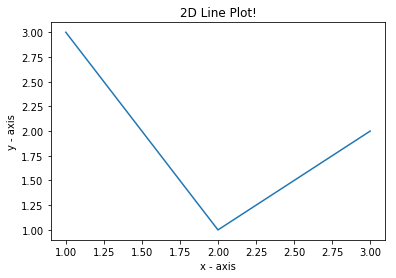## 2. Custom 2D Plot

a custom 2D plot is a plot which can be enhanced graphically
1. import matplotlib.pyplot as plt
2.
3. # x axis values
4. x = [1,2,3,4,5,6]
5. # corresponding y axis values
6. y = [2,4,1,5,2,6]
7.
8. # plotting the points
9. plt.plot(x, y, color='green', linestyle='dashed', linewidth = 3,
10.         marker='o', markerfacecolor='blue', markersize=12)
11.
12. # setting x and y axis range
13. plt.ylim(1,8)
14. plt.xlim(1,8)
15.
16. # naming the x axis
17. plt.xlabel('x - axis')
18. # naming the y axis
19. plt.ylabel('y - axis')
20.
21. # giving a title to my graph
22. plt.title('Custom Plots!')
23.
24. # function to show the plot
25. plt.show()
Output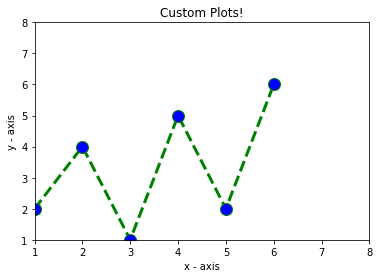## 3. Bar 2D Chart

A bar chart or bar graph is a chart or graph that presents categorical data with rectangular bars with heights or lengths proportional to the values that they represent. The bars can be plotted vertically or horizontally. A vertical bar chart is sometimes called a line graph.
1. import matplotlib.pyplot as plt
2.
3. # x-coordinates of left sides of bars
4. left = 
5.
6. # heights of bars
7. height = 
8.
9. # labels for bars
10. tick_label = ['one''two''three''four''five']
11.
12. # plotting a bar chart
13. plt.bar(left, height, tick_label = tick_label,
14.         width = 0.8, color = ['blue''green''red','yellow','black'])
15.
16. # naming the x-axis
17. plt.xlabel('x - axis')
18. # naming the y-axis
19. plt.ylabel('y - axis')
20. # plot title
21. plt.title('bar chart!')
22.
23. # function to show the plot
24. plt.show()
Output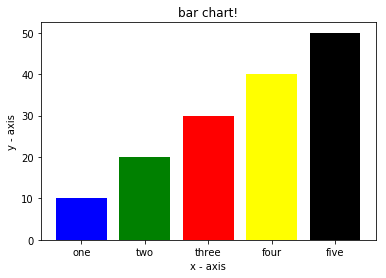## 4. Histogram 2D Plot

A histogram is an accurate representation of the distribution of numerical data. It is an estimate of the probability distribution of a continuous variable and was first introduced by Karl Pearson. It differs from a bar graph, in the sense that a bar graph relates two variables, but a histogram relates only one.
1. import matplotlib.pyplot as plt
2.
3. # frequencies
4. ages = [2,5,70,40,30,45,50,45,43,40,44,
5.         60,7,13,57,18,90,77,32,21,20,40]
6.
7. # setting the ranges and no. of intervals
8. range = (0100)
9. bins = 5
10.
11. # plotting a histogram
12. plt.hist(ages, bins, range, color ='red',
13.         histtype = 'bar', rwidth = 0.8)
14.
15. # x-axis label
16. plt.xlabel('age')
17. # frequency label
18. plt.ylabel('No. of people')
19. # plot title
20. plt.title('My histogram')
21.
22. # function to show the plot
23. plt.show()
Output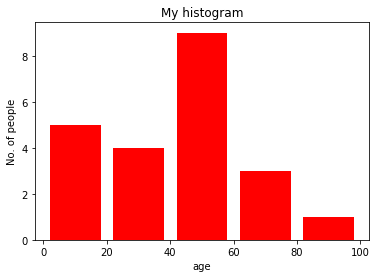## 5. Scatter 2D Plot

A scatter plot is a type of plot or mathematical diagram using Cartesian coordinates to display values for typically two variables for a set of data. If the points are coded, one additional variable can be displayed
1. import matplotlib.pyplot as plt
2.
3. # x-axis values
4. x = [2,4,5,7,6,8,9,11,12,12]
5. # y-axis values
6. y = [1,2,3,4,5,6,7,8,9,10]
7.
8. # plotting points as a scatter plot
9. plt.scatter(x, y, label= "stars", color= "green",
10.             marker= "x", s=30)
11.
12. # x-axis label
13. plt.xlabel('x - axis')
14. # frequency label
15. plt.ylabel('y - axis')
16. # plot title
17. plt.title('Scatter plot!')
18. # showing legend
19. plt.legend()
20.
21. # function to show the plot
22. plt.show()
Output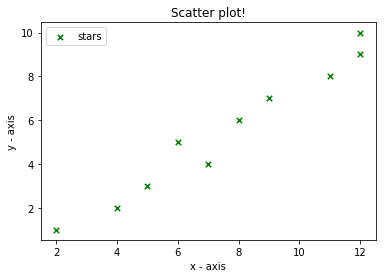## 6. Pie-Chart 2D Plot

A pie chart is a circular statistical graphic, which is divided into slices to illustrate numerical proportions. In a pie chart, the arc length of each slice is proportional to the quantity it represents.
1. import matplotlib.pyplot as plt
2.
3. # defining labels
4. activities = ['eat''sleep''work''play']
5.
6. # portion covered by each label
7. slices = 
8.
9. # color for each label
10. colors = ['r''y''g''b']
11.
12. # plotting the pie chart
13. plt.pie(slices, labels = activities, colors=colors,
14.         startangle=90, shadow = True, explode = (0.300.10),
15.         radius = 1.2, autopct = '%1.1f%%')
16.
17. # plotting legend
18. plt.legend()
19.
20. # showing the plot
21. plt.show()
Output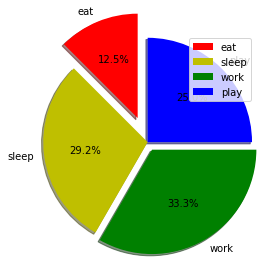## Plotting 3D Graphs

Toolkits are collections of application-specific functions that extend Matplotlib.

### mplot3d

The mplot3d toolkit adds simple 3D plotting capabilities to matplotlib by supplying an axes object that can create a 2D projection of a 3D scene. The resulting graph will have the same look and feel as regular 2D plots.

## 1. Line 3D Plot

A line chart or line plot or line graph or curve chart is a type of chart that displays information as a series of data points called 'markers' connected by straight line segments. It is a basic type of chart common in many fields.
1. # This import registers the 3D projection but is otherwise unused.
2. from mpl_toolkits.mplot3d import Axes3D  #F401 unused import
3.
4. import numpy as np
5. import matplotlib.pyplot as plt
6.
7.
8. plt.rcParams['legend.fontsize'] = 10
9.
10. fig = plt.figure()
11. ax = fig.gca(projection='3d')
12.
13. # Prepare arrays x, y, z
14. theta = np.linspace(-4 * np.pi, 4 * np.pi, 100)
15. z = np.linspace(-22100)
16. r = z**2 + 1
17. x = r * np.sin(theta)
18. y = r * np.cos(theta)
19.
20. ax.plot(x, y, z, label='parametric curve')
21. ax.legend()
22.
23. plt.show()
Output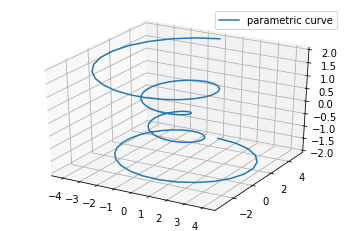## 2. Scatter 3D Plot

A scatter plot is a type of plot or mathematical diagram using Cartesian coordinates to display values for typically two variables for a set of data. If the points are coded, one additional variable can be displayed
1. # This import registers the 3D projection but is otherwise unused.
2. from mpl_toolkits.mplot3d import Axes3D  # noqa: F401 unused import
3.
4. import matplotlib.pyplot as plt
5. import numpy as np
6.
7. # Fixing random state for reproducibility
8. np.random.seed(19680801)
9.
10.
11. def randrange(n, vmin, vmax):
12.     '''''
13.     Helper function to make an array of random numbers having shape (n, )
14.     with each number distributed Uniform(vmin, vmax).
15.     '''
16.     return (vmax - vmin)*np.random.rand(n) + vmin
17.
18. fig = plt.figure()
20.
21. n = 100
22.
23. # For each set of style and range settings, plot n random points in the box
24. # defined by x in [23, 32], y in [0, 100], z in [zlow, zhigh].
25. for m, zlow, zhigh in [('o', -50, -25), ('^', -30, -5)]:
26.     xs = randrange(n, 2332)
27.     ys = randrange(n, 0100)
28.     zs = randrange(n, zlow, zhigh)
29.     ax.scatter(xs, ys, zs, marker=m)
30.
31. ax.set_xlabel('X Label')
32. ax.set_ylabel('Y Label')
33. ax.set_zlabel('Z Label')
34.
35. plt.show()
Output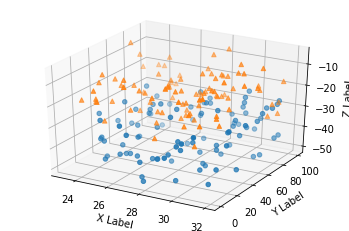## 3. WireFrame 3D Plot

Wireframe plot takes a grid of values and projects it onto the specified three-dimensional surface, and can make the resulting three-dimensional forms quite easy to visualize.
1. from mpl_toolkits.mplot3d import axes3d
2. import matplotlib.pyplot as plt
3.
4.
5. fig = plt.figure()
7.
8. # Grab some test data.
9. X, Y, Z = axes3d.get_test_data(0.05)
10.
11. # Plot a basic wireframe.
12. ax.plot_wireframe(X, Y, Z, rstride=10, cstride=10)
13.
14. plt.show()
Output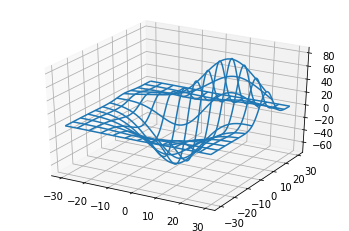## 4. Surface 3D Plot

Surface plots are diagrams of three-dimensional data. Rather than showing the individual data points, surface plots show a functional relationship between a designated dependent variable (Y), and two independent variables (X and Z). The plot is a companion plot to the contour plot.
1. # This import registers the 3D projection but is otherwise unused.
2. from mpl_toolkits.mplot3d import Axes3D  # F401 unused import
3.
4. import matplotlib.pyplot as plt
5. from matplotlib import cm
6. from matplotlib.ticker import LinearLocator, FormatStrFormatter
7. import numpy as np
8.
9.
10. fig = plt.figure()
11. ax = fig.gca(projection='3d')
12.
13. # Make data.
14. X = np.arange(-550.5)
15. Y = np.arange(-550.5)
16. X, Y = np.meshgrid(X, Y)
17. R = np.sqrt(X**2 + Y**2)
18. Z = np.sin(R)
19.
20. # Plot the surface.
21. surf = ax.plot_surface(X, Y, Z, cmap=cm.coolwarm,
22.                        linewidth=0, antialiased=False)
23.
24. # Customize the z axis.
25. ax.set_zlim(-1.011.01)
26. ax.zaxis.set_major_locator(LinearLocator(10))
27. ax.zaxis.set_major_formatter(FormatStrFormatter('%.02f'))
28.
29. # Add a color bar that maps values to colors.
30. fig.colorbar(surf, shrink=0.5, aspect=5)
31.
32. plt.show()
Output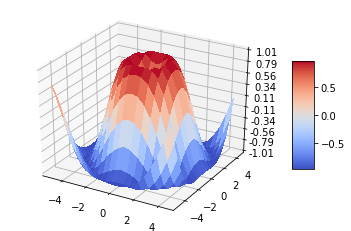## 5. Tri-Surface 3D Plot

A triangulation of a compact surface is a finite collection of triangles that cover the surface in such a way that every point on the surface is in a triangle, and the intersection of any two triangles is either void, a common edge, or a common vertex. A triangulated is called tri-surface.
1. from mpl_toolkits.mplot3d import Axes3D
2. import matplotlib.pyplot as plt
3. import numpy as np
4.
5.
7. n_angles = 16
8.
9. # Make radii and angles spaces (radius r=0 omitted to eliminate duplication).
11. angles = np.linspace(02*np.pi, n_angles, endpoint=False)
12.
13. # Repeat all angles for each radius.
14. angles = np.repeat(angles[..., np.newaxis], n_radii, axis=1)
15.
16. # Convert polar (radii, angles) coords to cartesian (x, y) coords.
17. # (0, 0) is manually added at this stage,  so there will be no duplicate
18. # points in the (x, y) plane.
21.
22. # Compute z to make the pringle surface.
23. z = np.sin(-x*y)
24.
25. fig = plt.figure()
26. ax = fig.gca(projection='3d')
27.
28. ax.plot_trisurf(x, y, z, linewidth=0.2, antialiased=True)
29.
30. plt.show()
Output## 6. Contour 3D Plot

A contour line of a function of two variables is a curve along which the function has a constant value so that the curve joins points of equal value. It is a plane section of the three-dimensional graph of the function f parallel to the plane. A contour plot is a graphical technique for representing a 3-dimensional surface by plotting constant z slices, called contours, on a 2-dimensional format. That is, given a value for z, lines are drawn for connecting the (x,y) coordinates where that z value occurs.
1. from mpl_toolkits.mplot3d import axes3d
2. import matplotlib.pyplot as plt
3. from matplotlib import cm
4.
5. fig = plt.figure()
6. ax = fig.gca(projection='3d')
7. X, Y, Z = axes3d.get_test_data(0.005)
8. cset = ax.contour(X, Y, Z, extend3d=True, cmap=cm.coolwarm)
9. ax.clabel(cset, fontsize=9, inline=1)
10.
11. plt.show()
Output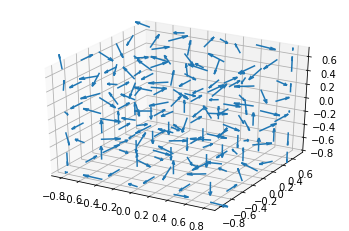## 7. Filled Contour 3D Plot

This is a type of contour plot where each 3D artist is filled
1. from mpl_toolkits.mplot3d import axes3d
2. import matplotlib.pyplot as plt
3. from matplotlib import cm
4.
5. fig = plt.figure()
6. ax = fig.gca(projection='3d')
7. X, Y, Z = axes3d.get_test_data(0.005)
8. cset = ax.contourf(X, Y, Z, cmap=cm.coolwarm)
9. ax.clabel(cset, fontsize=9, inline=1)
10.
11. plt.show()
Output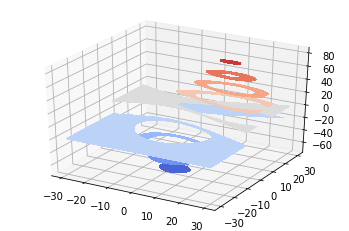## 8. Polygon 3D Plot

A Polygon plot is a type of 3D plot, where the artists can be of different shapes and sizes with no restriction on the shape or size of any artist
1. from mpl_toolkits.mplot3d import Axes3D
2. from matplotlib.collections import PolyCollection
3. import matplotlib.pyplot as plt
4. from matplotlib import colors as mcolors
5. import numpy as np
6.
7.
8. fig = plt.figure()
9. ax = fig.gca(projection='3d')
10.
11.
12. def cc(arg):
13.     return mcolors.to_rgba(arg, alpha=0.9)
14.
15. xs = np.arange(0100.2)
16. verts = []
17. zs = [0.01.02.03.0]
18. for z in zs:
19.     ys = np.random.rand(len(xs))
20.     ys, ys[-1] = 00
21.     verts.append(list(zip(xs, ys)))
22.
23. poly = PolyCollection(verts, facecolors=[cc('r'), cc('g'), cc('b'),
24.                                          cc('y')])
25. poly.set_alpha(0.9)
27.
28. ax.set_xlabel('X')
29. ax.set_xlim3d(010)
30. ax.set_ylabel('Y')
31. ax.set_ylim3d(-14)
32. ax.set_zlabel('Z')
33. ax.set_zlim3d(01)
34.
35. plt.show()
Output## 9. Bar 3D Plot

A bar chart or bar graph is a chart or graph that presents categorical data with rectangular bars with heights or lengths proportional to the values that they represent. The bars can be plotted vertically or horizontally. A vertical bar chart is sometimes called a line graph.
1. from mpl_toolkits.mplot3d import Axes3D
2. import matplotlib.pyplot as plt
3. import numpy as np
4.
5. fig = plt.figure()
7. for c, z in zip(['r''g''b''y'], ):
8.     xs = np.arange(100)
9.     ys = np.random.rand(100)
10.
11.     # You can provide either a single color or an array. To demonstrate this,
12.     # the first bar of each set will be colored cyan.
13.     cs = [c] * len(xs)
14.     cs = 'c'
15.     ax.bar(xs, ys, zs=z, zdir='y', color=cs, alpha=0.8)
16.
17. ax.set_xlabel('X')
18. ax.set_ylabel('Y')
19. ax.set_zlabel('Z')
20.
21. plt.show()
Output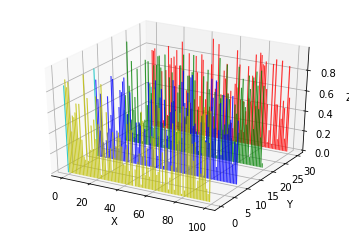## 10. Quiver 3D

A quiver plot displays velocity vectors as arrows with components (u,v) at the points (x,y) .
1. from mpl_toolkits.mplot3d import axes3d
2. import matplotlib.pyplot as plt
3. import numpy as np
4.
5. fig = plt.figure()
6. ax = fig.gca(projection='3d')
7.
8. # Make the grid
9. x, y, z = np.meshgrid(np.arange(-0.810.4),
10.                       np.arange(-0.810.3),
11.                       np.arange(-0.810.3))
12.
13. # Make the direction data for the arrows
14. u = np.sin(np.pi * x) * np.cos(np.pi * y) * np.cos(np.pi * z)
15. v = -np.cos(np.pi * x) * np.sin(np.pi * y) * np.cos(np.pi * z)
16. w = (np.sqrt(2.0 / 3.0) * np.cos(np.pi * x) * np.cos(np.pi * y) *
17.      np.sin(np.pi * z))
18.
19. ax.quiver(x, y, z, u, v, w, length=0.2, normalize=True)
20.
21. plt.show()
Output## Conclusion

In this chapter, we studied Python MatPlotLib. In the next chapter, we will learn about Python Seaborn.

Python Seaborn is a Python data visualization library based on Python MatPlotLib. It provides a high-level interface for drawing attractive and informative statistical graphics.
Next » Python Libraries for Machine Learning: Seaborn